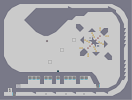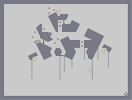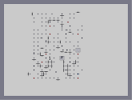### E.V.E.Hover over the thumbnail for a full-size version.

Author darkcrusader author:darkcrusader crap darkcrusader defecation featured feces n-art rated 2010-05-06 2011-09-18 5 by 38 people. \$E.V.E.#darkcrusader#n-art#00000000000000000000000000000000000000000000000000000000000000000000000000000000000000000000000000000000000000000000000000000000000000000000000000000000000000000000000000000000000000000000000000000000000000000000000000000000000000000000000000000000000000000000000000000000000000000000000000000000000000000000000000000000000000000000000000000000000000000000000000000000000000000000000000000000000000000000000000000000000000000000000000000000000000000000000000000000000000000000000000000000000000000000000000000000000000000000000000000000000000000000000000000000000000000000000000000000000000000000000000000000000000000000000000000000000000000000000000000000000000000000000000000000000000000000000000000000000000000|12^708,576!12^768,480!12^732,384!12^749,428!12^758,450!12^739,403!12^763,464!12^753,439!12^744,416!12^736,395!12^734,389!12^737,399!12^766,476!12^764,469!12^761,459!12^759,454!12^756,446!12^752,436!12^750,431!12^748,424!12^746,421!12^743,412!12^741,408!12^705,571!12^701,565!12^697,559!12^695,554!12^693,550!12^691,546!12^689,543!12^687,539!12^725,388!12^720,393!12^716,397!12^728,386!12^712,401!12^685,535!12^695,410!12^694,408!12^694,403!12^693,412!12^693,324!12^694,385!12^693,424!12^693,419!12^693,416!12^693,387!12^694,398!12^694,394!12^694,389!12^692,429!12^693,368!12^692,349!12^692,338!12^692,331!12^692,325!12^692,432!12^692,437!12^692,441!12^692,444!12^692,450!12^691,452!12^691,453!12^691,459!12^690,466!12^690,469!12^689,476!12^689,480!12^689,488!12^689,491!12^688,497!12^688,502!12^687,507!12^687,511!12^687,517!12^686,525!12^685,535!12^684,544!12^684,552!12^683,557!12^682,565!12^681,570!12^679,575!12^686,531!12^683,550!12^693,380!12^693,374!12^693,372!12^692,361!12^692,356!12^692,344!12^708,405!12^704,409!12^700,413!12^698,413!12^697,416!12^688,326!12^690,324!12^692,322!12^686,325!12^681,327!12^674,328!12^668,329!12^661,330!12^655,330!12^650,330!12^643,331!12^636,331!12^631,331!12^627,331!12^625,332!12^618,332!12^613,332!12^608,332!12^602,332!12^595,332!12^589,332!12^587,331!12^584,331!12^579,331!12^573,331!12^567,330!12^560,330!12^557,330!12^550,329!12^544,329!12^540,329!12^534,328!12^525,328!12^531,329!12^519,328!12^513,328!12^509,328!12^506,327!12^500,327!12^495,327!12^491,326!12^486,326!12^482,325!12^475,325!12^471,325!12^468,325!12^465,325!12^459,325!12^453,325!12^447,324!12^442,324!12^436,324!12^428,324!12^424,323!12^419,323!12^415,323!12^408,323!12^401,322!12^394,322!12^387,322!12^382,322!12^361,320!12^360,320!12^353,320!12^351,320!12^340,320!12^369,321!12^375,321!12^379,321!12^363,321!12^336,319!12^330,319!12^323,319!12^315,319!12^311,319!12^303,319!12^298,319!12^293,319!12^286,319!12^280,319!12^276,319!12^271,319!12^265,319!12^307,319!12^319,320!12^288,319!12^325,319!12^356,320!12^410,324!12^432,324!12^390,321!12^403,323!12^395,323!12^643,330!12^306,536!12^303,508!12^305,527!12^205,573!12^183,473!12^193,522!12^198,545!12^188,500!12^185,486!12^190,511!12^195,532!12^200,553!12^203,565!12^202,560!12^204,569!12^196,536!12^197,542!12^194,528!12^191,516!12^191,515!12^190,507!12^189,503!12^187,495!12^187,491!12^184,483!12^184,475!12^222,413!12^220,408!12^207,450!12^196,464!12^218,432!12^222,417!12^219,428!12^221,421!12^216,437!12^213,442!12^211,446!12^204,455!12^201,460!12^198,462!12^193,467!12^189,470!12^186,473!12^258,320!12^262,318!12^216,399!12^200,411!12^192,409!12^188,408!12^197,410!12^189,404!12^194,376!12^194,376!12^186,372!12^178,370!12^185,405!12^181,404!12^177,403!12^175,400!12^170,397!12^166,394!12^174,396!12^235,282!12^235,280!12^231,278!12^225,278!12^222,277!12^205,340!12^204,345!12^202,349!12^200,356!12^199,360!12^196,364!12^195,368!12^193,373!12^210,282!12^212,280!12^213,276!12^219,275!12^232,284!12^257,322!12^253,322!12^249,320!12^202,413!12^206,408!12^209,405!12^211,401!12^215,393!12^217,390!12^220,386!12^223,382!12^225,377!12^234,358!12^232,361!12^229,371!12^239,283!12^244,285!12^248,288!12^248,290!12^248,295!12^246,299!12^246,305!12^245,311!12^245,314!12^245,319!12^244,322!12^243,326!12^242,330!12^242,335!12^235,354!12^234,357!12^232,363!12^230,366!12^229,372!12^198,367!12^196,368!12^209,332!12^207,334!12^204,347!12^201,352!12^196,363!12^206,337!12^208,339!12^241,338!12^176,479!12^178,477!12^125,501!12^121,501!12^112,497!12^109,493!12^106,485!12^115,500!12^104,483!12^94,399!12^93,406!12^93,414!12^93,421!12^92,429!12^103,481!12^102,476!12^101,473!12^99,467!12^97,463!12^95,456!12^95,452!12^94,447!12^93,442!12^92,438!12^92,434!12^170,482!12^167,485!12^163,488!12^158,492!12^151,494!12^145,498!12^129,502!12^134,501!12^138,500!12^142,498!12^154,494!12^147,496!12^162,392!12^163,374!12^162,379!12^160,383!12^160,389!12^158,393!12^158,399!12^155,405!12^154,407!12^150,407!12^144,404!12^138,404!12^132,401!12^126,399!12^120,399!12^114,396!12^108,395!12^103,395!12^96,393!12^94,393!12^90,390!12^87,388!12^82,387!12^163,368!12^165,363!12^167,355!12^167,350!12^168,344!12^168,338!12^168,333!12^168,327!12^169,319!12^169,311!12^169,304!12^168,295!12^168,289!12^166,280!12^166,275!12^163,264!12^161,261!12^165,269!12^163,265!12^168,323!12^169,320!12^169,313!12^169,307!12^168,301!12^168,294!12^168,290!12^168,285!12^167,360!12^166,363!12^165,367!12^163,372!12^163,378!12^160,392!12^158,400!12^212,273!12^208,276!12^206,273!12^203,275!12^199,271!12^195,268!12^197,262!12^199,269!12^207,275!12^203,273!12^203,269!12^159,257!12^156,254!12^152,248!12^148,244!12^143,240!12^193,258!12^193,250!12^193,245!12^191,234!12^191,231!12^190,227!12^171,254!12^175,254!12^177,254!12^180,254!12^183,254!12^187,254!12^193,255!12^192,238!12^167,225!12^161,216!12^156,209!12^164,221!12^78,385!12^71,379!12^69,374!12^68,366!12^68,356!12^69,348!12^70,341!12^72,316!12^74,305!12^77,292!12^78,283!12^80,272!12^83,257!12^87,246!12^91,232!12^96,218!12^99,204!12^108,201!12^112,205!12^102,200!12^115,208!12^118,212!12^132,227!12^135,231!12^131,226!12^127,221!12^124,218!12^122,216!12^141,239!12^137,232!12^139,236!12^137,211!12^140,217!12^140,221!12^143,227!12^144,229!12^142,231!12^133,204!12^130,201!12^124,197!12^121,196!12^119,196!12^156,209!12^152,205!12^149,201!12^156,193!12^158,190!12^161,188!12^166,183!12^168,180!12^166,175!12^163,168!12^158,162!12^153,155!12^151,152!12^142,142!12^137,138!12^134,136!12^129,132!12^124,127!12^121,126!12^118,124!12^117,125!12^115,130!12^114,136!12^113,142!12^113,145!12^113,153!12^111,158!12^111,164!12^116,193!12^114,187!12^112,183!12^111,177!12^111,167!12^110,173!12^96,211!12^94,221!12^93,225!12^91,230!12^91,235!12^89,238!12^88,243!12^86,246!12^84,251!12^84,256!12^83,260!12^82,263!12^81,266!12^79,272!12^78,279!12^77,283!12^77,291!12^76,297!12^74,301!12^73,307!12^72,316!12^69,341!12^67,352!12^67,358!12^67,364!12^71,312!12^69,338!12^69,335!12^70,331!12^70,327!12^70,324!12^70,318!12^70,315!12^188,223!12^184,223!12^181,223!12^177,223!12^174,223!12^171,223!12^170,223!12^167,224!12^167,228!12^165,233!12^165,235!12^165,241!12^165,242!12^165,248!12^164,250!12^164,255!12^168,255!12^150,199!12^150,196!12^156,159!12^161,165!12^165,172!12^150,150!12^146,146!12^145,145!12^217,267!12^217,260!12^217,254!12^216,249!12^195,213!12^198,217!12^214,243!12^208,230!12^205,226!12^211,236!12^202,222!12^201,220!12^186,208!12^188,207!12^211,235!12^213,240!12^181,217!12^182,213!12^183,209!12^184,205!12^185,203!12^189,205!12^191,208!12^714,187!12^713,183!12^712,176!12^711,171!12^710,167!12^708,162!12^707,156!12^705,152!12^674,88!12^692,117!12^703,148!12^702,143!12^701,137!12^700,133!12^697,128!12^696,123!12^694,119!12^691,114!12^688,109!12^686,105!12^683,101!12^680,98!12^678,93!12^676,90!12^670,83!12^667,79!12^664,75!12^658,70!12^656,68!12^653,63!12^649,60!12^647,58!12^639,51!12^637,48!12^633,44!12^629,40!12^624,36!12^621,32!12^617,30!12^614,27!12^609,25!12^644,55!12^639,49!12^660,71!12^311,27!12^291,48!12^244,252!12^370,316!12^376,317!12^385,319!12^381,320!12^345,321!12^349,312!12^344,311!12^340,311!12^335,309!12^331,309!12^327,308!12^322,306!12^257,267!12^255,265!12^250,259!12^246,255!12^281,287!12^303,298!12^270,278!12^317,304!12^311,302!12^299,295!12^296,295!12^293,293!12^288,291!12^287,289!12^309,301!12^278,286!12^274,283!12^273,281!12^268,277!12^264,273!12^261,270!12^241,248!12^238,245!12^236,242!12^233,238!12^232,236!12^221,199!12^225,219!12^221,187!12^221,196!12^230,232!12^228,227!12^226,223!12^223,216!12^222,212!12^222,209!12^221,207!12^221,203!12^220,198!12^220,191!12^307,30!12^303,34!12^300,38!12^295,42!12^293,45!12^289,48!12^285,53!12^282,56!12^278,61!12^274,65!12^271,69!12^269,73!12^265,78!12^220,185!12^220,181!12^220,173!12^221,167!12^221,162!12^223,158!12^224,149!12^226,144!12^229,138!12^230,133!12^232,127!12^234,123!12^237,118!12^242,107!12^245,101!12^249,97!12^253,92!12^239,113!12^256,88!12^260,83!12^570,93!12^486,73!12^366,316!12^362,315!12^358,315!12^351,313!12^450,75!12^531,79!12^598,291!12^659,252!12^671,215!12^390,89!12^316,25!12^655,257!12^650,263!12^647,266!12^643,269!12^558,303!12^317,266!12^343,280!12^403,296!12^373,290!12^624,280!12^578,298!12^530,306!12^491,306!12^511,307!12^425,300!12^456,304!12^473,306!12^669,234!12^669,194!12^620,124!12^590,103!12^552,85!12^469,74!12^509,75!12^431,78!12^409,83!12^419,80!12^399,85!12^377,93!12^345,108!12^354,103!12^337,112!12^327,119!12^332,115!12^316,129!12^324,121!12^319,126!12^310,134!12^313,131!12^311,263!12^306,260!12^301,258!12^295,255!12^290,251!12^285,247!12^283,244!12^279,240!12^338,277!12^333,274!12^327,272!12^324,270!12^320,267!12^371,289!12^366,288!12^363,288!12^358,286!12^353,284!12^348,282!12^277,237!12^276,234!12^274,230!12^274,226!12^273,219!12^273,209!12^273,213!12^273,205!12^274,200!12^275,195!12^277,191!12^279,186!12^285,167!12^280,182!12^282,178!12^284,172!12^288,164!12^293,155!12^296,150!12^299,144!12^290,159!12^303,140!12^306,137!12^397,295!12^391,295!12^386,293!12^381,293!12^377,291!12^420,300!12^416,300!12^415,299!12^412,299!12^409,297!12^407,297!12^452,304!12^449,304!12^445,303!12^443,303!12^440,303!12^438,302!12^434,302!12^432,302!12^430,301!12^459,305!12^462,305!12^465,306!12^469,306!12^477,306!12^483,306!12^505,307!12^500,307!12^495,307!12^492,307!12^489,307!12^485,307!12^479,307!12^515,307!12^519,307!12^523,307!12^526,307!12^535,306!12^540,306!12^543,306!12^546,305!12^549,305!12^552,304!12^574,300!12^569,301!12^556,303!12^701,257!12^690,275!12^710,233!12^715,206!12^716,203!12^715,199!12^715,196!12^715,193!12^715,211!12^714,216!12^713,221!12^711,228!12^713,224!12^703,252!12^704,247!12^707,243!12^708,238!12^699,262!12^697,264!12^694,271!12^689,279!12^692,274!12^686,283!12^682,288!12^679,291!12^675,295!12^671,299!12^666,301!12^662,304!12^657,306!12^654,308!12^647,311!12^645,313!12^638,315!12^632,319!12^614,326!12^611,326!12^599,330!12^625,321!12^618,325!12^620,323!12^564,302!12^583,297!12^591,295!12^596,293!12^618,283!12^614,286!12^604,289!12^637,273!12^632,277!12^627,279!12^609,288!12^586,297!12^667,239!12^662,246!12^665,242!12^671,232!12^671,227!12^672,221!12^671,210!12^671,203!12^669,197!12^668,189!12^661,170!12^667,184!12^666,180!12^663,175!12^659,166!12^657,162!12^654,158!12^651,154!12^646,147!12^642,143!12^637,139!12^650,151!12^635,136!12^632,133!12^627,129!12^623,125!12^617,120!12^613,117!12^610,115!12^342,109!12^348,105!12^359,101!12^364,98!12^371,96!12^384,91!12^395,86!12^405,83!12^413,82!12^424,79!12^436,77!12^442,76!12^438,76!12^597,107!12^594,105!12^605,112!12^602,109!12^585,99!12^580,98!12^574,95!12^565,90!12^560,88!12^556,86!12^550,83!12^545,81!12^539,80!12^535,79!12^527,77!12^523,76!12^516,75!12^514,75!12^503,73!12^498,73!12^490,73!12^479,73!12^474,73!12^468,73!12^464,73!12^458,75!12^453,75!12^447,75!12^445,209!12^429,223!12^360,204!12^327,159!12^344,161!12^368,167!12^396,173!12^621,196!12^614,180!12^607,181!12^617,200!12^613,205!12^608,210!12^604,214!12^601,216!12^598,218!12^414,176!12^406,175!12^400,173!12^393,173!12^389,173!12^385,171!12^378,171!12^376,169!12^372,167!12^364,165!12^359,164!12^355,164!12^350,163!12^346,161!12^341,161!12^336,159!12^330,158!12^381,171!12^374,168!12^447,206!12^443,213!12^440,217!12^434,221!12^424,225!12^418,227!12^411,227!12^405,227!12^395,224!12^385,220!12^392,223!12^400,226!12^379,217!12^374,214!12^369,211!12^362,206!12^357,202!12^350,197!12^343,190!12^339,188!12^336,182!12^330,176!12^327,171!12^327,158!12^325,161!12^324,166!12^332,178!12^347,193!12^354,199!12^338,186!12^620,179!12^624,179!12^628,180!12^629,183!12^625,190!12^622,193!12^585,229!12^589,226!12^593,224!12^597,221!12^579,232!12^524,223!12^520,221!12^553,187!12^604,183!12^598,183!12^594,183!12^587,184!12^582,184!12^578,185!12^574,185!12^565,186!12^557,186!12^569,186!12^560,186!12^549,187!12^542,186!12^534,186!12^527,187!12^518,187!12^522,186!12^509,190!12^511,186!12^515,186!12^448,200!12^450,195!12^450,190!12^451,186!12^450,183!12^446,183!12^440,183!12^437,182!12^432,180!12^428,179!12^424,179!12^418,179!12^413,177!12^408,175!12^574,234!12^568,236!12^563,236!12^556,236!12^508,195!12^508,200!12^521,221!12^516,218!12^512,214!12^509,210!12^509,207!12^509,202!12^552,236!12^546,235!12^541,233!12^534,230!12^530,228!12^523,222!12^168,390!12^162,390!12^162,384!12^168,378!12^168,372!12^168,360!12^168,366!12^174,366!12^180,366!12^186,384!12^180,378!12^180,390!12^186,396!12^180,402!12^174,396!12^192,366!12^186,366!12^192,360!12^186,360!12^180,360!12^174,360!12^198,354!12^192,354!12^186,354!12^180,354!12^174,354!12^168,354!12^174,348!12^180,348!12^186,348!12^192,348!12^198,348!12^198,342!12^192,342!12^186,342!12^180,342!12^174,342!12^198,336!12^204,336!12^192,336!12^198,318!12^210,318!12^204,324!12^192,330!12^204,312!12^210,306!12^216,312!12^204,300!12^216,300!12^210,294!12^222,294!12^216,288!12^222,282!12^210,282!12^186,336!12^186,330!12^180,330!12^180,336!12^174,336!12^174,330!12^174,324!12^174,318!12^174,312!12^180,312!12^186,312!12^192,312!12^192,324!12^186,324!12^180,324!12^180,318!12^186,318!12^198,306!12^198,282!12^198,276!12^186,306!12^180,306!12^174,306!12^174,300!12^180,300!12^186,300!12^192,294!12^186,294!12^186,288!12^192,288!12^180,294!12^174,294!12^174,288!12^180,288!12^180,282!12^186,282!12^192,282!12^192,276!12^192,270!12^192,264!12^186,276!12^180,276!12^174,282!12^174,276!12^186,270!12^180,270!12^174,270!12^168,270!12^216,282!12^186,264!12^180,264!12^174,264!12^168,258!12^180,258!12^192,258!12^186,258!12^174,258!12^168,264!12^174,276!12^138,144!12^132,144!12^126,144!12^120,150!12^114,156!12^114,162!12^114,168!12^120,174!12^150,174!12^156,162!12^156,168!12^162,180!12^150,192!12^162,174!12^156,168!12^156,174!12^150,186!12^114,180!12^120,180!12^114,174!12^129,135!12^123,136!12^119,140!12^121,132!12^144,172!12^142,167!12^138,162!12^134,159!12^131,155!12^127,149!12^150,168!12^150,162!12^150,156!12^144,156!12^144,162!12^138,156!12^132,150!12^138,150!12^144,150!12^132,174!12^138,168!12^126,168!12^132,162!12^120,162!12^126,156!12^150,180!12^114,169!12^121,174!12^118,152!12^125,150!12^114,170!12^122,178!12^125,183!12^130,185!12^136,185!12^145,186!12^140,186!12^146,183!12^149,179!12^153,177!12^120,189!12^124,191!12^129,193!12^144,194!12^137,194!12^130,194!12^152,191!12^157,188!12^159,184!12^138,180!12^126,180!12^144,174!12^138,198!12^144,198!12^132,198!12^138,204!12^144,204!12^144,210!12^150,210!12^156,216!12^150,204!12^144,216!12^150,216!12^156,222!12^150,222!12^156,246!12^156,240!12^156,234!12^162,246!12^162,240!12^162,234!12^162,252!12^150,240!12^150,234!12^144,234!12^150,228!12^156,228!12^162,228!12^144,222!12^156,222!12^228,408!12^234,402!12^222,402!12^228,396!12^240,396!12^252,396!12^246,402!12^264,396!12^276,396!12^258,390!12^270,390!12^282,390!12^276,384!12^282,378!12^270,378!12^264,384!12^252,384!12^246,390!12^234,390!12^222,390!12^228,384!12^240,384!12^246,378!12^234,378!12^258,378!12^276,372!12^264,372!12^270,366!12^252,372!12^240,372!12^258,366!12^246,366!12^234,366!12^210,330!12^228,288!12^234,288!12^240,288!12^232,294!12^229,299!12^227,302!12^226,308!12^223,311!12^221,317!12^218,323!12^214,329!12^214,333!12^214,329!12^215,325!12^218,322!12^240,294!12^240,306!12^234,300!12^240,300!12^234,294!12^234,306!12^228,312!12^228,306!12^234,312!12^240,312!12^228,318!12^234,318!12^234,324!12^228,324!12^222,324!12^228,330!12^234,330!12^222,336!12^222,330!12^228,336!12^228,342!12^222,348!12^222,354!12^222,360!12^216,330!12^216,336!12^222,342!12^216,342!12^210,342!12^210,354!12^210,348!12^198,330!12^216,348!12^216,354!12^216,360!12^210,360!12^204,360!12^210,348!12^204,354!12^216,366!12^216,372!12^210,378!12^210,372!12^210,366!12^204,366!12^204,372!12^204,378!12^198,378!12^198,372!12^198,384!12^198,390!12^198,402!12^198,402!12^198,396!12^192,390!12^192,378!12^192,402!12^192,396!12^192,384!12^204,402!12^204,384!12^204,390!12^204,390!12^204,396!12^210,396!12^210,384!12^210,384!12^192,300!12^198,294!12^204,288!12^174,384!12^168,384!12^174,372!12^264,360!12^252,360!12^240,360!12^258,354!12^246,354!12^233,332!12^231,337!12^229,341!12^227,346!12^227,350!12^226,356!12^224,361!12^221,364!12^220,370!12^215,376!12^213,382!12^211,386!12^208,391!12^238,316!12^236,319!12^240,342!12^238,349!12^252,348!12^258,342!12^246,342!12^258,474!12^282,486!12^288,492!12^294,498!12^276,492!12^282,498!12^288,504!12^300,504!12^294,510!12^294,522!12^300,516!12^300,528!12^294,534!12^300,540!12^294,546!12^300,552!12^294,558!12^306,558!12^306,546!12^300,564!12^294,570!12^306,570!12^300,576!12^282,510!12^276,504!12^276,516!12^276,528!12^276,540!12^276,552!12^276,564!12^276,576!12^282,522!12^282,534!12^282,546!12^282,558!12^282,570!12^270,486!12^264,492!12^258,570!12^258,558!12^258,546!12^258,534!12^258,522!12^258,510!12^258,498!12^258,486!12^264,480!12^252,480!12^252,492!12^252,504!12^252,516!12^252,528!12^252,540!12^252,552!12^252,564!12^252,576!12^228,576!12^240,576!12^234,570!12^246,570!12^240,564!12^246,558!12^240,552!12^246,546!12^240,540!12^246,534!12^240,528!12^246,522!12^240,516!12^246,510!12^234,558!12^234,546!12^246,498!12^216,576!12^204,576!12^204,564!12^204,552!12^204,540!12^204,528!12^204,516!12^204,504!12^204,492!12^204,480!12^210,474!12^210,462!12^216,456!12^216,444!12^210,450!12^204,468!12^198,474!12^192,480!12^198,486!12^186,486!12^192,492!12^198,498!12^192,504!12^198,510!12^198,522!12^198,534!12^222,570!12^228,564!12^228,552!12^228,540!12^234,534!12^234,522!12^234,510!12^240,504!12^240,492!12^246,486!12^246,474!12^252,468!12^270,474!12^276,480!12^294,486!12^288,486!12^282,480!12^288,480!12^294,480!12^282,474!12^282,468!12^282,456!12^282,462!12^282,444!12^282,450!12^294,474!12^294,468!12^294,462!12^288,468!12^288,474!12^288,462!12^288,456!12^288,450!12^282,438!12^282,432!12^282,426!12^282,420!12^282,414!12^276,456!12^276,450!12^276,438!12^276,444!12^276,426!12^276,432!12^276,420!12^276,414!12^276,408!12^282,402!12^288,426!12^288,420!12^288,432!12^288,432!12^288,438!12^288,444!12^294,456!12^294,444!12^294,438!12^294,450!12^300,462!12^300,468!12^300,474!12^300,480!12^300,486!12^300,492!12^306,498!12^306,486!12^306,480!12^306,492!12^300,456!12^294,432!12^288,396!12^264,348!12^270,354!12^276,360!12^270,426!12^270,420!12^270,414!12^270,408!12^270,402!12^264,420!12^264,414!12^264,408!12^264,402!12^258,402!12^252,402!12^240,408!12^228,420!12^228,426!12^222,432!12^222,438!12^222,444!12^222,426!12^222,450!12^222,456!12^216,462!12^216,468!12^216,474!12^222,462!12^210,480!12^210,486!12^210,492!12^210,498!12^216,480!12^216,486!12^210,504!12^222,468!12^228,432!12^234,414!12^234,420!12^210,510!12^210,522!12^210,516!12^276,468!12^270,462!12^264,468!12^258,462!12^264,456!12^270,450!12^258,450!12^288,408!12^234,426!12^264,444!12^270,438!12^264,432!12^258,438!12^258,426!12^258,414!12^252,408!12^246,414!12^240,420!12^240,432!12^234,438!12^234,408!12^240,402!12^228,414!12^246,396!12^258,396!12^252,420!12^246,426!12^252,432!12^246,438!12^234,450!12^228,444!12^252,444!12^240,444!12^246,450!12^252,456!12^240,456!12^228,456!12^234,462!12^246,462!12^240,468!12^240,480!12^234,474!12^228,468!12^222,474!12^228,480!12^234,486!12^240,498!12^240,510!12^234,516!12^234,528!12^234,540!12^228,546!12^228,558!12^222,576!12^222,564!12^228,492!12^234,498!12^222,486!12^222,498!12^216,492!12^228,504!12^216,504!12^222,510!12^228,516!12^216,516!12^222,522!12^228,528!12^216,528!12^222,534!12^210,534!12^216,540!12^222,546!12^210,546!12^216,552!12^210,558!12^222,558!12^216,564!12^204,564!12^210,570!12^252,336!12^252,324!12^264,324!12^264,336!12^276,324!12^288,324!12^288,336!12^300,336!12^300,324!12^312,324!12^312,336!12^276,348!12^288,348!12^300,348!12^312,348!12^600,576!12^600,564!12^600,552!12^600,540!12^600,528!12^600,516!12^588,516!12^588,504!12^588,492!12^588,480!12^588,468!12^588,456!12^588,444!12^576,444!12^576,432!12^576,420!12^576,408!12^576,396!12^576,396!12^576,384!12^576,372!12^576,360!12^576,348!12^576,336!12^564,336!12^564,348!12^552,348!12^552,336!12^540,336!12^540,348!12^528,348!12^528,336!12^516,336!12^516,348!12^504,348!12^588,336!12^588,348!12^588,360!12^588,372!12^588,384!12^588,396!12^588,408!12^588,420!12^588,432!12^600,444!12^600,456!12^600,468!12^600,480!12^600,492!12^600,504!12^612,516!12^612,528!12^612,540!12^612,552!12^612,564!12^612,576!12^624,564!12^624,576!12^588,576!12^588,564!12^588,552!12^588,540!12^588,528!12^576,528!12^576,516!12^576,504!12^576,492!12^576,480!12^576,468!12^576,456!12^576,540!12^576,552!12^576,564!12^576,576!12^504,336!12^492,336!12^492,348!12^480,348!12^480,336!12^468,336!12^468,348!12^456,348!12^456,336!12^444,336!12^444,348!12^432,348!12^432,336!12^420,336!12^420,348!12^408,348!12^324,324!12^324,336!12^324,348!12^336,348!12^336,336!12^336,324!12^348,324!12^348,336!12^348,348!12^360,348!12^360,336!12^372,336!12^372,348!12^384,348!12^396,348!12^384,336!12^396,336!12^408,336!12^360,324!12^372,324!12^384,324!12^396,324!12^288,360!12^300,360!12^312,360!12^324,360!12^336,360!12^348,360!12^360,360!12^372,360!12^384,360!12^396,360!12^408,360!12^420,360!12^420,360!12^432,360!12^444,360!12^444,360!12^456,360!12^468,360!12^480,360!12^492,360!12^270,444!12^264,438!12^270,432!12^264,426!12^258,432!12^270,456!12^276,462!12^276,474!12^252,432!12^252,426!12^258,420!12^258,408!12^252,414!12^246,408!12^240,414!12^246,420!12^240,426!12^234,432!12^246,432!12^222,438!12^228,438!12^228,450!12^228,462!12^216,498!12^216,510!12^204,510!12^204,522!12^204,534!12^294,492!12^300,498!12^504,360!12^516,360!12^528,360!12^540,360!12^552,360!12^564,360!12^564,372!12^552,372!12^540,372!12^528,372!12^516,372!12^504,372!12^492,372!12^480,372!12^468,372!12^456,372!12^444,372!12^432,372!12^420,372!12^408,372!12^396,372!12^384,372!12^372,372!12^360,372!12^348,372!12^336,372!12^324,372!12^312,372!12^300,372!12^288,372!12^300,384!12^288,384!12^300,396!12^300,408!12^300,420!12^300,432!12^300,444!12^312,456!12^312,468!12^312,480!12^312,492!12^312,504!12^312,516!12^312,528!12^312,540!12^312,552!12^312,564!12^312,576!12^324,576!12^324,564!12^324,552!12^324,540!12^324,528!12^324,516!12^324,504!12^324,492!12^324,480!12^324,468!12^324,456!12^324,444!12^312,444!12^312,432!12^312,420!12^312,408!12^312,396!12^312,384!12^324,384!12^336,384!12^348,384!12^360,384!12^372,384!12^372,396!12^360,396!12^348,396!12^336,396!12^324,396!12^324,408!12^324,420!12^324,432!12^336,432!12^336,444!12^336,456!12^336,480!12^336,468!12^336,492!12^336,504!12^336,516!12^336,528!12^336,552!12^336,564!12^336,576!12^336,540!12^600,336!12^612,336!12^624,336!12^636,336!12^600,348!12^612,348!12^648,336!12^564,384!12^552,384!12^540,384!12^528,384!12^516,384!12^504,384!12^492,384!12^480,384!12^468,384!12^456,384!12^444,384!12^432,384!12^420,384!12^564,396!12^552,396!12^540,396!12^528,396!12^516,396!12^504,396!12^492,396!12^480,396!12^468,396!12^456,396!12^444,396!12^432,396!12^420,396!12^408,396!12^408,384!12^396,384!12^396,396!12^384,396!12^384,384!12^336,408!12^336,420!12^348,408!12^348,420!12^348,432!12^348,444!12^348,456!12^348,468!12^348,480!12^348,492!12^348,504!12^348,516!12^348,528!12^348,540!12^348,552!12^348,564!12^348,576!12^360,576!12^360,564!12^372,564!12^372,576!12^564,576!12^564,564!12^564,552!12^564,540!12^564,528!12^564,516!12^552,516!12^540,516!12^540,504!12^552,504!12^564,504!12^564,492!12^552,492!12^540,492!12^540,480!12^552,480!12^564,480!12^564,468!12^552,468!12^540,468!12^540,456!12^528,456!12^516,456!12^516,444!12^516,432!12^504,432!12^492,432!12^492,420!12^492,408!12^504,408!12^504,420!12^516,420!12^516,408!12^528,408!12^528,420!12^528,432!12^528,444!12^540,444!12^552,444!12^552,456!12^564,456!12^564,444!12^564,444!12^564,432!12^552,432!12^540,432!12^540,420!12^552,420!12^564,420!12^564,408!12^552,408!12^540,408!12^480,408!12^468,408!12^456,408!12^444,408!12^432,408!12^420,408!12^408,408!12^396,408!12^372,408!12^384,408!12^360,408!12^360,420!12^372,420!12^384,420!12^396,420!12^408,420!12^420,420!12^432,420!12^444,420!12^456,420!12^468,420!12^480,420!12^480,432!12^468,432!12^456,432!12^444,432!12^432,432!12^420,432!12^408,432!12^396,432!12^384,432!12^372,432!12^360,432!12^360,444!12^360,456!12^372,444!12^384,444!12^396,444!12^408,444!12^420,444!12^432,444!12^444,444!12^456,444!12^468,444!12^480,444!12^492,444!12^504,444!12^504,456!12^492,456!12^480,456!12^468,456!12^456,456!12^444,456!12^432,456!12^420,456!12^408,456!12^396,456!12^384,456!12^372,456!12^360,468!12^360,480!12^360,492!12^360,504!12^360,528!12^360,516!12^360,540!12^360,564!12^360,552!12^372,552!12^372,540!12^372,528!12^372,516!12^372,504!12^372,492!12^372,480!12^372,468!12^552,528!12^540,528!12^540,540!12^552,540!12^552,552!12^540,552!12^540,564!12^552,564!12^552,576!12^540,576!12^528,468!12^516,468!12^516,480!12^528,480!12^528,492!12^516,492!12^504,492!12^504,480!12^504,468!12^384,468!12^396,468!12^396,480!12^384,480!12^384,492!12^396,492!12^408,492!12^408,480!12^408,468!12^384,504!12^396,504!12^408,504!12^384,516!12^396,516!12^408,516!12^384,528!12^396,528!12^408,528!12^384,540!12^396,540!12^396,540!12^408,540!12^384,552!12^396,552!12^408,552!12^384,564!12^396,564!12^408,564!12^408,576!12^396,576!12^384,576!12^420,468!12^432,468!12^432,480!12^420,480!12^420,492!12^432,492!12^444,492!12^444,480!12^444,468!12^456,468!12^456,480!12^456,492!12^468,492!12^468,480!12^468,468!12^480,468!12^480,480!12^480,492!12^492,492!12^492,480!12^492,468!12^528,504!12^516,504!12^504,504!12^492,504!12^480,504!12^468,504!12^456,504!12^444,504!12^432,504!12^420,504!12^420,516!12^432,516!12^444,516!12^456,516!12^468,516!12^480,516!12^492,516!12^504,516!12^516,516!12^528,516!12^528,528!12^516,528!12^504,528!12^492,528!12^480,528!12^468,528!12^456,528!12^444,528!12^432,528!12^420,528!12^420,540!12^432,540!12^444,540!12^456,540!12^468,540!12^480,540!12^492,540!12^504,540!12^528,540!12^516,540!12^516,552!12^528,552!12^528,564!12^516,564!12^528,576!12^516,576!12^504,576!12^504,564!12^504,552!12^492,552!12^480,552!12^480,564!12^492,564!12^492,576!12^480,576!12^468,552!12^456,552!12^456,564!12^468,564!12^468,576!12^456,576!12^444,576!12^432,576!12^432,564!12^444,564!12^444,552!12^432,552!12^420,552!12^420,564!12^420,576!12^318,342!12^294,342!12^342,342!12^294,366!12^318,366!12^342,366!12^366,366!12^366,342!12^294,390!12^318,390!12^342,390!12^318,414!12^294,414!12^318,438!12^318,462!12^600,360!12^624,360!12^624,384!12^600,384!12^600,408!12^624,408!12^600,432!12^624,432!12^624,456!12^624,480!12^624,504!12^624,528!12^648,432!12^648,456!12^648,480!12^648,504!12^648,528!12^648,552!12^624,552!12^648,576!12^672,576!12^672,552!12^648,360!12^702,426!12^708,432!12^696,420!12^714,438!12^714,450!12^720,456!12^720,468!12^720,480!12^726,486!12^726,498!12^726,510!12^732,516!12^732,528!12^732,540!12^738,546!12^738,558!12^744,564!12^744,576!12^738,570!12^732,576!12^732,564!12^732,552!12^726,546!12^726,534!12^726,522!12^720,516!12^720,504!12^720,492!12^714,486!12^714,474!12^714,462!12^708,456!12^708,444!12^702,438!12^696,432!12^696,420!12^696,444!12^702,450!12^696,456!12^702,462!12^708,468!12^696,468!12^702,474!12^708,480!12^696,480!12^702,486!12^708,492!12^714,498!12^696,492!12^702,498!12^708,504!12^714,510!12^720,528!12^714,522!12^708,516!12^702,510!12^696,504!12^690,498!12^690,510!12^696,516!12^702,522!12^708,528!12^714,534!12^720,540!12^726,558!12^726,570!12^720,576!12^714,570!12^720,564!12^708,564!12^714,558!12^720,552!12^714,546!12^708,552!12^702,558!12^696,552!12^702,546!12^708,540!12^702,534!12^696,540!12^690,534!12^696,528!12^690,522!12^696,426!12^702,432!12^708,438!12^714,444!12^714,456!12^714,468!12^720,462!12^720,474!12^720,486!12^726,492!12^720,498!12^726,504!12^726,516!12^732,522!12^732,534!12^732,546!12^738,552!12^738,540!12^738,564!12^744,570!12^738,576!12^132,252!12^132,264!12^132,240!12^132,276!12^132,288!12^132,300!12^120,300!12^120,312!12^120,324!12^120,336!12^120,348!12^108,348!12^120,264!12^120,276!12^120,288!12^120,252!12^108,336!12^108,324!12^108,312!12^96,336!12^96,348!12^96,360!12^96,384!12^96,372!12^84,372!12^84,384!12^108,384!12^108,372!12^108,360!12^120,360!12^120,372!12^120,384!12^132,384!12^132,396!12^132,372!12^132,360!12^132,348!12^132,336!12^132,324!12^132,312!12^144,252!12^144,264!12^144,276!12^144,288!12^144,300!12^144,312!12^108,300!12^108,288!12^108,276!12^108,264!12^108,252!12^96,324!12^96,312!12^96,300!12^120,240!12^120,228!12^144,324!12^84,360!12^144,336!12^144,348!12^144,360!12^144,372!12^144,396!12^144,384!12^156,384!12^156,360!12^156,372!12^156,348!12^156,336!12^156,324!12^156,312!12^156,300!12^156,288!12^156,276!12^156,264!12^108,420!12^108,432!12^108,408!12^108,444!12^120,456!12^120,468!12^120,480!12^120,492!12^120,444!12^120,432!12^108,456!12^120,420!12^120,408!12^132,408!12^144,408!12^156,408!12^168,408!12^180,408!12^204,408!12^192,420!12^180,420!12^168,420!12^156,420!12^144,420!12^132,420!12^132,432!12^132,444!12^132,468!12^132,480!12^132,492!12^144,492!12^144,480!12^144,468!12^144,456!12^132,456!12^144,444!12^144,432!12^156,432!12^168,432!12^180,432!12^192,432!12^204,420!12^216,420!12^204,432!12^216,432!12^204,444!12^192,444!12^180,444!12^168,444!12^156,444!12^156,456!12^156,468!12^156,480!12^168,480!12^168,468!12^168,456!12^180,456!12^180,468!12^192,456!12^252,234!12^258,228!12^252,222!12^252,210!12^258,216!12^258,204!12^252,198!12^246,204!12^246,216!12^246,228!12^240,210!12^240,222!12^234,216!12^240,234!12^234,228!12^246,240!12^252,246!12^258,252!12^264,258!12^258,240!12^264,246!12^270,252!12^276,246!12^270,240!12^264,234!12^270,228!12^264,222!12^270,216!12^264,210!12^270,204!12^264,198!12^258,192!12^234,204!12^234,192!12^240,198!12^240,186!12^246,192!12^252,186!12^234,180!12^246,180!12^240,174!12^240,162!12^234,168!12^246,156!12^246,168!12^252,174!12^252,162!12^252,150!12^246,144!12^252,126!12^264,186!12^258,180!12^270,180!12^270,192!12^258,168!12^264,174!12^276,174!12^270,168!12^276,162!12^264,162!12^258,156!12^270,156!12^264,150!12^258,144!12^252,138!12^258,132!12^264,138!12^270,144!12^276,150!12^282,156!12^288,150!12^282,144!12^276,138!12^270,132!12^264,126!12^312,42!12^306,48!12^300,54!12^294,60!12^288,66!12^288,78!12^282,84!12^276,90!12^270,96!12^264,102!12^258,120!12^264,114!12^270,108!12^276,102!12^282,96!12^288,90!12^294,84!12^294,72!12^306,60!12^300,66!12^312,54!12^318,48!12^318,36!12^324,30!12^324,42!12^330,36!12^336,30!12^330,24!12^342,24!12^630,318!12^480,324!12^270,264!12^276,270!12^282,276!12^300,282!12^306,288!12^318,288!12^294,276!12^324,294!12^336,294!12^342,300!12^354,300!12^360,306!12^372,306!12^378,312!12^390,312!12^402,312!12^396,318!12^408,306!12^396,306!12^408,318!12^384,306!12^390,300!12^378,300!12^366,300!12^372,294!12^402,300!12^360,288!12^360,294!12^348,294!12^354,288!12^342,288!12^330,288!12^336,282!12^324,282!12^330,276!12^312,282!12^318,276!12^306,276!12^312,270!12^300,270!12^288,270!12^294,264!12^306,264!12^282,264!12^276,258!12^288,258!12^282,252!12^270,120!12^276,126!12^282,132!12^288,138!12^294,144!12^294,132!12^288,126!12^282,120!12^276,114!12^282,108!12^300,126!12^288,114!12^300,114!12^294,120!12^294,108!12^288,102!12^294,96!12^300,78!12^300,90!12^300,102!12^300,138!12^306,132!12^306,120!12^306,108!12^306,96!12^306,84!12^306,72!12^312,66!12^318,60!12^324,54!12^330,48!12^336,42!12^342,36!12^348,30!12^354,24!12^366,24!12^378,24!12^390,24!12^402,24!12^414,24!12^426,24!12^438,24!12^510,60!12^510,72!12^516,66!12^504,66!12^492,66!12^498,60!12^504,54!12^498,48!12^456,30!12^444,30!12^432,30!12^420,30!12^408,30!12^396,30!12^384,30!12^372,30!12^360,30!12^342,48!12^342,60!12^330,60!12^336,54!12^336,66!12^324,66!12^318,72!12^312,78!12^324,78!12^330,84!12^318,84!12^330,72!12^336,78!12^342,72!12^342,84!12^312,90!12^330,96!12^516,54!12^522,60!12^522,72!12^528,66!12^534,72!12^540,66!12^546,72!12^552,78!12^540,78!12^480,66!12^486,60!12^492,54!12^480,54!12^486,48!12^480,42!12^468,42!12^474,60!12^474,48!12^468,54!12^468,66!12^456,66!12^462,60!12^456,54!12^462,48!12^456,42!12^450,36!12^438,36!12^426,36!12^324,90!12^318,96!12^312,102!12^318,108!12^312,114!12^318,120!12^324,114!12^324,102!12^330,108!12^336,102!12^336,90!12^342,96!12^348,90!12^348,102!12^354,96!12^354,84!12^348,78!12^354,72!12^348,66!12^354,60!12^348,54!12^354,48!12^348,42!12^354,36!12^360,42!12^366,36!12^372,42!12^378,48!12^384,54!12^372,54!12^366,48!12^360,54!12^384,42!12^378,36!12^432,42!12^444,42!12^450,48!12^438,48!12^426,48!12^432,54!12^444,54!12^450,60!12^438,60!12^426,60!12^378,60!12^366,60!12^390,60!12^390,48!12^390,36!12^360,66!12^372,66!12^384,66!12^390,72!12^378,72!12^366,72!12^360,78!12^372,78!12^384,78!12^378,84!12^390,84!12^366,84!12^360,90!12^372,90!12^396,66!12^396,54!12^396,42!12^402,36!12^414,36!12^420,42!12^408,42!12^402,48!12^414,48!12^408,54!12^420,54!12^402,60!12^414,60!12^444,66!12^432,66!12^420,66!12^408,66!12^402,72!12^414,72!12^426,72!12^438,72!12^450,72!12^396,78!12^408,78!12^648,294!12^654,300!12^642,288!12^630,288!12^636,294!12^648,282!12^636,282!12^642,300!12^648,306!12^630,300!12^636,306!12^642,312!12^624,294!12^618,288!12^624,282!12^414,312!12^420,306!12^420,318!12^426,312!12^432,306!12^432,318!12^438,312!12^444,306!12^444,318!12^450,312!12^456,318!12^456,306!12^462,312!12^468,318!12^474,312!12^480,318!12^486,312!12^492,324!12^492,318!12^498,312!12^504,318!12^498,324!12^516,318!12^510,312!12^510,324!12^522,324!12^528,318!12^522,312!12^534,312!12^540,318!12^534,324!12^546,324!12^546,312!12^552,318!12^558,324!12^564,318!12^570,324!12^576,318!12^582,324!12^588,318!12^594,324!12^600,318!12^606,324!12^612,318!12^624,318!12^630,312!12^618,312!12^624,306!12^612,306!12^618,300!12^612,294!12^606,300!12^606,312!12^594,312!12^600,306!12^588,306!12^582,312!12^570,312!12^558,312!12^576,306!12^564,306!12^594,300!12^600,294!12^258,108!12^240,150!12^246,132!12^468,30!12^480,30!12^492,30!12^492,42!12^462,36!12^504,30!12^504,42!12^516,42!12^516,30!12^528,30!12^528,42!12^528,54!12^540,54!12^540,42!12^552,42!12^552,54!12^552,66!12^564,54!12^564,66!12^564,78!12^576,66!12^576,78!12^576,90!12^588,78!12^588,90!12^600,90!12^600,102!12^612,102!12^612,114!12^624,114!12^624,126!12^588,66!12^576,54!12^600,78!12^660,282!12^660,294!12^660,270!12^648,270!12^672,282!12^672,294!12^672,270!12^660,258!12^672,258!12^684,270!12^684,258!12^684,246!12^696,246!12^696,258!12^366,390!12^390,366!12^390,342!12^342,414!12^342,438!12^342,462!12^318,486!12^318,510!12^270,342!12^318,162!12^318,156!12^324,156!12^330,156!12^324,168!12^330,174!12^330,168!12^336,162!12^342,168!12^336,174!12^348,174!12^342,180!12^354,180!12^354,168!12^348,186!12^360,186!12^360,174!12^366,180!12^372,174!12^378,180!12^372,186!12^354,192!12^366,192!12^378,192!12^360,198!12^372,198!12^366,204!12^378,204!12^372,210!12^384,210!12^390,216!12^402,216!12^414,216!12^396,222!12^408,222!12^420,222!12^426,216!12^438,216!12^432,210!12^438,204!12^438,192!12^444,198!12^444,186!12^432,186!12^420,186!12^408,186!12^396,186!12^384,186!12^384,174!12^390,180!12^402,180!12^390,192!12^402,192!12^414,192!12^426,192!12^432,198!12^420,198!12^408,198!12^396,198!12^384,198!12^390,204!12^402,204!12^414,204!12^426,204!12^420,210!12^408,210!12^396,210!12^510,198!12^516,198!12^522,192!12^522,204!12^510,204!12^516,210!12^528,210!12^528,198!12^534,192!12^534,204!12^534,216!12^522,216!12^534,228!12^528,222!12^540,222!12^540,210!12^540,198!12^546,192!12^546,204!12^546,216!12^546,228!12^552,222!12^552,234!12^552,210!12^552,198!12^558,192!12^558,204!12^558,216!12^558,228!12^564,222!12^564,210!12^564,198!12^570,192!12^570,204!12^570,216!12^570,228!12^576,222!12^576,210!12^576,198!12^582,192!12^582,204!12^582,216!12^582,228!12^588,222!12^588,210!12^588,198!12^588,186!12^594,192!12^594,204!12^594,216!12^600,210!12^600,198!12^600,186!12^606,192!12^606,204!12^612,198!12^612,186!12^618,192!12^624,186!12^618,180!12^564,300!12^564,294!12^564,288!12^564,282!12^564,276!12^564,270!12^564,264!12^564,258!12^564,246!12^564,240!12^564,252!12^564,180!12^564,168!12^564,174!12^564,162!12^564,156!12^564,144!12^564,150!12^564,138!12^564,126!12^564,120!12^564,114!12^564,108!12^564,102!12^564,96!12^564,90!12^564,132!12^396,168!12^396,162!12^402,150!12^396,156!12^396,150!12^396,138!12^396,144!12^396,132!12^396,126!12^396,120!12^396,108!12^396,102!12^396,96!12^396,90!12^396,114!12^396,228!12^396,234!12^396,240!12^396,252!12^396,246!12^396,264!12^396,258!12^396,270!12^396,276!12^396,282!12^396,288!12^390,162!12^390,168!12^390,156!12^390,150!12^390,144!12^390,132!12^390,138!12^390,126!12^390,120!12^390,114!12^390,108!12^390,102!12^390,96!12^384,96!12^384,102!12^372,102!12^378,102!12^378,96!12^366,102!12^354,108!12^360,108!12^366,108!12^372,108!12^384,108!12^378,108!12^348,108!12^414,102!12^414,108!12^414,114!12^414,120!12^420,120!12^426,120!12^426,114!12^432,120!12^438,120!12^438,114!12^438,108!12^438,102!12^450,120!12^450,114!12^450,108!12^450,102!12^462,102!12^462,108!12^462,114!12^462,120!12^474,102!12^474,114!12^474,108!12^474,120!12^480,120!12^486,120!12^498,102!12^498,114!12^498,108!12^498,120!12^504,120!12^510,120!12^522,102!12^522,108!12^522,114!12^522,120!12^414,96!12^438,96!12^450,96!12^462,96!12^456,96!12^456,108!12^474,96!12^498,96!12^522,96!12^528,96!12^534,96!12^528,108!12^528,120!12^534,120!12^480,198!12^474,198!12^480,192!12^486,198!12^480,204!12^444,246!12^432,246!12^438,246!12^426,246!12^426,258!12^426,252!12^432,258!12^426,264!12^426,270!12^432,270!12^438,270!12^444,270!12^474,246!12^474,258!12^474,252!12^474,264!12^480,270!12^486,264!12^486,258!12^486,252!12^486,246!12^516,246!12^516,252!12^516,258!12^516,264!12^516,270!12^528,270!12^534,270!12^522,270!12^522,258!12^522,246!12^528,246!12^534,246!12^384,114!12^378,114!12^366,114!12^372,114!12^360,114!12^354,114!12^348,114!12^342,114!12^336,114!12^384,120!12^378,120!12^378,126!12^384,126!12^372,132!12^372,126!12^372,120!12^378,132!12^384,132!12^384,168!12^384,162!12^378,162!12^372,162!12^366,162!12^360,162!12^354,162!12^336,156!12^342,156!12^348,156!12^354,156!12^360,156!12^366,156!12^372,156!12^378,156!12^384,156!12^384,150!12^378,150!12^372,150!12^360,150!12^366,150!12^360,150!12^354,150!12^348,150!12^342,150!12^336,150!12^330,150!12^324,150!12^318,150!12^402,156!12^402,162!12^402,168!12^414,168!12^378,168!12^408,168!12^420,174!12^426,174!12^432,174!12^414,174!12^438,174!12^444,174!12^450,174!12^450,180!12^444,180!12^438,180!12^456,174!12^456,186!12^456,180!12^456,192!12^456,198!12^456,204!12^456,210!12^456,216!12^456,222!12^456,228!12^450,228!12^444,228!12^438,228!12^432,228!12^426,228!12^450,222!12^450,216!12^450,210!12^450,204!12^444,222!12^438,222!12^444,216!12^420,228!12^414,228!12^408,228!12^402,228!12^396,228!12^390,228!12^384,228!12^378,228!12^372,228!12^366,228!12^354,228!12^348,228!12^360,228!12^384,222!12^378,222!12^390,222!12^372,222!12^366,222!12^360,222!12^354,222!12^348,222!12^348,216!12^354,216!12^360,216!12^366,216!12^372,216!12^366,210!12^360,210!12^354,210!12^348,210!12^354,204!12^354,204!12^348,204!12^348,198!12^342,228!12^336,228!12^330,228!12^324,228!12^318,228!12^318,222!12^318,216!12^318,210!12^318,198!12^318,192!12^318,186!12^318,180!12^318,174!12^318,168!12^318,204!12^342,222!12^324,222!12^324,174!12^324,180!12^324,186!12^324,192!12^324,198!12^330,186!12^330,192!12^330,180!12^336,186!12^336,192!12^342,192!12^342,198!12^336,210!12^330,210!12^336,216!12^330,204!12^342,204!12^330,216!12^330,216!12^324,210!12^324,204!12^324,216!12^330,222!12^336,222!12^342,216!12^342,210!12^336,204!12^336,198!12^330,198!12^408,150!12^408,156!12^408,162!12^414,162!12^420,162!12^420,168!12^426,168!12^432,168!12^438,168!12^444,168!12^450,168!12^456,168!12^456,162!12^450,162!12^444,162!12^438,162!12^432,162!12^426,162!12^414,156!12^414,150!12^420,150!12^420,156!12^426,156!12^426,150!12^432,150!12^432,156!12^438,156!12^438,150!12^444,150!12^444,156!12^450,156!12^450,150!12^456,150!12^450,156!12^456,156!12^504,198!12^504,192!12^504,186!12^504,180!12^504,174!12^504,162!12^504,168!12^504,156!12^504,150!12^510,150!12^516,150!12^528,150!12^522,150!12^504,204!12^504,210!12^504,210!12^504,216!12^504,222!12^504,228!12^504,234!12^510,234!12^522,234!12^516,234!12^528,234!12^534,234!12^540,234!12^546,234!12^552,234!12^558,234!12^564,234!12^570,234!12^576,234!12^588,234!12^594,234!12^522,228!12^516,228!12^510,228!12^528,228!12^516,222!12^510,222!12^510,216!12^582,234!12^576,240!12^564,240!12^570,240!12^558,240!12^552,240!12^552,240!12^546,240!12^540,240!12^540,240!12^582,240!12^588,240!12^594,240!12^594,228!12^600,228!12^600,234!12^600,240!12^600,222!12^588,228!12^528,240!12^534,240!12^522,240!12^516,240!12^510,240!12^504,240!12^504,252!12^504,246!12^510,246!12^510,252!12^504,252!12^504,258!12^510,258!12^504,264!12^504,270!12^510,270!12^510,264!12^522,264!12^528,264!12^528,258!12^528,252!12^522,252!12^528,252!12^534,252!12^534,258!12^534,264!12^540,270!12^546,264!12^552,258!12^558,252!12^540,252!12^546,258!12^552,264!12^558,270!12^552,246!12^546,246!12^546,276!12^552,276!12^552,270!12^546,270!12^540,264!12^540,258!12^552,252!12^546,252!12^540,246!12^558,246!12^558,258!12^558,264!12^558,276!12^558,282!12^552,282!12^546,282!12^546,288!12^552,288!12^558,288!12^546,294!12^552,294!12^558,294!12^558,300!12^552,300!12^546,300!12^540,300!12^534,300!12^528,300!12^522,300!12^516,300!12^510,300!12^504,300!12^504,294!12^504,288!12^504,282!12^504,276!12^510,276!12^510,282!12^516,282!12^516,276!12^540,276!12^540,282!12^534,282!12^534,276!12^534,294!12^540,294!12^516,294!12^510,294!12^522,276!12^528,276!12^540,288!12^534,288!12^528,294!12^522,294!12^528,282!12^522,282!12^522,288!12^528,288!12^516,288!12^510,288!12^570,246!12^570,252!12^570,258!12^576,258!12^576,252!12^576,252!12^576,246!12^582,246!12^582,252!12^582,258!12^570,282!12^576,282!12^582,282!12^582,288!12^582,294!12^576,294!12^570,294!12^570,288!12^576,288!12^576,270!12^582,264!12^570,276!12^570,264!12^582,276!12^582,270!12^576,276!12^570,270!12^576,264!12^588,246!12^594,252!12^606,258!12^600,258!12^600,246!12^588,258!12^594,264!12^594,270!12^606,270!12^618,258!12^624,258!12^630,264!12^630,270!12^618,270!12^600,264!12^594,258!12^588,252!12^594,246!12^600,252!12^588,264!12^588,276!12^588,270!12^588,282!12^588,288!12^588,294!12^594,294!12^594,288!12^594,282!12^594,276!12^600,276!12^600,282!12^600,288!12^600,270!12^606,276!12^606,282!12^612,276!12^612,282!12^618,276!12^624,276!12^624,270!12^624,264!12^618,264!12^612,270!12^606,264!12^612,264!12^612,258!12^630,258!12^606,252!12^612,252!12^624,252!12^618,252!12^606,246!12^612,246!12^618,246!12^624,246!12^630,246!12^630,252!12^636,264!12^636,258!12^636,252!12^636,246!12^636,270!12^642,270!12^642,258!12^642,252!12^642,246!12^642,264!12^648,258!12^648,252!12^648,246!12^654,246!12^654,252!12^660,246!12^660,252!12^606,216!12^606,222!12^606,228!12^606,234!12^606,240!12^612,240!12^618,240!12^624,240!12^630,240!12^636,240!12^612,234!12^612,228!12^624,228!12^618,228!12^618,234!12^618,222!12^660,240!12^660,234!12^666,234!12^654,234!12^660,228!12^654,210!12^654,204!12^648,204!12^654,198!12^660,204!12^666,204!12^660,198!12^654,192!12^654,186!12^654,180!12^654,174!12^654,168!12^654,162!12^666,198!12^666,192!12^660,192!12^660,186!12^660,180!12^660,174!12^636,210!12^630,210!12^630,216!12^630,204!12^630,198!12^630,192!12^624,210!12^618,210!12^612,210!12^624,204!12^624,198!12^624,192!12^618,204!12^642,240!12^648,240!12^654,240!12^624,234!12^612,222!12^612,216!12^654,192!12^642,192!12^636,192!12^648,192!12^648,198!12^636,204!12^636,198!12^636,192!12^642,192!12^642,198!12^666,210!12^666,216!12^666,222!12^666,228!12^660,210!12^660,216!12^660,222!12^654,222!12^654,216!12^654,228!12^648,234!12^642,234!12^636,234!12^630,234!12^630,228!12^630,222!12^624,222!12^624,216!12^618,216!12^636,228!12^642,228!12^648,228!12^648,222!12^636,222!12^642,222!12^636,216!12^642,216!12^648,216!12^648,210!12^642,210!12^642,204!12^648,186!12^642,186!12^636,186!12^630,186!12^636,180!12^642,180!12^648,180!12^648,174!12^648,168!12^648,162!12^648,156!12^648,150!12^642,150!12^636,150!12^624,150!12^630,150!12^618,150!12^606,150!12^612,144!12^612,150!12^594,150!12^600,150!12^588,150!12^582,150!12^570,150!12^576,150!12^564,150!12^558,150!12^552,150!12^540,150!12^534,150!12^540,150!12^546,150!12^516,192!12^510,180!12^510,174!12^510,162!12^516,156!12^510,168!12^510,156!12^522,156!12^516,162!12^516,168!12^516,174!12^516,180!12^522,162!12^528,156!12^534,156!12^540,156!12^546,156!12^552,156!12^558,156!12^558,162!12^570,162!12^570,156!12^576,156!12^582,156!12^588,156!12^594,156!12^600,156!12^606,156!12^606,162!12^612,168!12^612,174!12^612,162!12^612,156!12^618,156!12^642,156!12^636,156!12^624,156!12^624,156!12^630,156!12^618,162!12^642,162!12^606,168!12^606,174!12^600,162!12^570,180!12^570,174!12^570,168!12^576,162!12^636,162!12^642,168!12^642,174!12^636,174!12^636,168!12^630,162!12^624,162!12^618,168!12^618,174!12^624,174!12^630,174!12^630,168!12^624,168!12^594,162!12^588,162!12^582,162!12^576,168!12^582,168!12^588,168!12^588,168!12^594,168!12^600,168!12^600,174!12^594,174!12^594,180!12^600,180!12^588,180!12^582,180!12^576,180!12^576,174!12^582,174!12^582,174!12^588,174!12^558,180!12^552,180!12^546,180!12^540,180!12^534,180!12^528,180!12^522,180!12^522,174!12^552,174!12^558,174!12^552,162!12^528,162!12^528,174!12^540,174!12^540,162!12^522,168!12^558,168!12^546,168!12^534,168!12^528,168!12^534,174!12^540,168!12^546,174!12^552,168!12^546,162!12^546,162!12^534,162!12^474,192!12^474,204!12^486,204!12^486,192!12^486,192!12^492,198!12^498,198!12^498,192!12^486,192!12^492,204!12^498,204!12^468,192!12^468,198!12^468,204!12^462,204!12^462,198!12^462,192!12^486,192!12^492,192!12^618,144!12^624,144!12^630,144!12^636,144!12^642,144!12^612,144!12^606,144!12^600,144!12^594,144!12^588,144!12^582,144!12^576,144!12^570,144!12^570,138!12^570,132!12^570,126!12^570,120!12^570,114!12^570,108!12^570,102!12^570,96!12^630,138!12^624,132!12^618,132!12^624,138!12^630,132!12^618,132!12^618,126!12^612,126!12^612,120!12^606,120!12^606,114!12^600,114!12^618,138!12^612,132!12^600,126!12^612,138!12^606,132!12^606,126!12^600,120!12^594,114!12^594,120!12^588,114!12^594,108!12^588,108!12^588,102!12^582,102!12^582,108!12^576,102!12^588,120!12^588,126!12^594,126!12^576,132!12^582,132!12^582,138!12^576,138!12^588,138!12^576,126!12^582,114!12^600,132!12^606,138!12^594,132!12^600,138!12^588,138!12^594,138!12^588,132!12^582,126!12^582,120!12^576,120!12^576,114!12^576,108!12^558,144!12^552,138!12^540,132!12^540,126!12^540,138!12^540,144!12^546,126!12^552,126!12^558,126!12^546,132!12^546,138!12^546,144!12^552,144!12^558,138!12^558,132!12^552,132!12^534,144!12^528,144!12^534,138!12^534,132!12^522,144!12^528,138!12^534,126!12^522,132!12^528,132!12^522,138!12^516,138!12^516,144!12^510,144!12^528,126!12^528,114!12^534,114!12^540,114!12^546,114!12^552,114!12^558,114!12^558,120!12^552,120!12^540,120!12^546,120!12^534,108!12^540,108!12^546,108!12^552,108!12^558,108!12^558,102!12^552,102!12^546,102!12^540,102!12^534,102!12^528,102!12^540,96!12^546,96!12^552,96!12^558,96!12^558,90!12^552,90!12^546,90!12^540,90!12^534,90!12^528,90!12^522,90!12^522,84!12^534,84!12^540,84!12^546,84!12^528,84!12^516,114!12^510,108!12^504,102!12^516,108!12^516,102!12^510,102!12^504,96!12^510,96!12^516,96!12^516,90!12^510,90!12^504,90!12^498,90!12^492,96!12^492,102!12^486,108!12^480,114!12^468,126!12^474,132!12^480,138!12^486,144!12^492,150!12^498,156!12^486,102!12^480,108!12^468,120!12^468,114!12^468,102!12^468,108!12^456,102!12^468,96!12^480,96!12^480,102!12^486,96!12^516,84!12^510,84!12^504,84!12^498,84!12^498,78!12^504,78!12^510,78!12^516,78!12^522,78!12^492,90!12^486,90!12^480,90!12^474,90!12^468,90!12^462,90!12^456,90!12^450,90!12^450,84!12^456,84!12^462,84!12^474,84!12^480,84!12^486,84!12^492,84!12^492,78!12^486,78!12^480,78!12^474,78!12^468,78!12^468,84!12^462,78!12^456,78!12^450,78!12^486,114!12^492,114!12^492,120!12^492,114!12^492,108!12^504,108!12^504,114!12^510,114!12^516,120!12^522,126!12^516,126!12^516,132!12^510,138!12^510,132!12^510,126!12^504,126!12^504,132!12^504,138!12^504,144!12^498,150!12^498,144!12^498,138!12^498,126!12^498,132!12^492,144!12^492,138!12^486,138!12^480,132!12^480,126!12^474,126!12^486,126!12^492,132!12^486,132!12^492,126!12^456,120!12^456,114!12^444,120!12^444,114!12^444,108!12^444,102!12^444,96!12^444,90!12^444,84!12^444,78!12^432,114!12^432,108!12^426,108!12^420,108!12^420,114!12^420,102!12^420,96!12^426,96!12^426,102!12^432,102!12^432,90!12^432,96!12^438,90!12^438,84!12^432,84!12^420,84!12^426,84!12^414,84!12^408,84!12^426,90!12^420,90!12^414,90!12^408,90!12^402,90!12^414,126!12^420,126!12^432,126!12^426,126!12^438,126!12^444,126!12^450,126!12^456,126!12^462,126!12^468,132!12^462,132!12^450,132!12^456,132!12^444,132!12^438,132!12^432,132!12^426,132!12^420,132!12^414,132!12^474,138!12^474,144!12^474,150!12^474,162!12^474,156!12^474,168!12^474,174!12^486,174!12^492,174!12^498,180!12^498,174!12^498,186!12^480,174!12^480,168!12^480,162!12^486,162!12^486,168!12^498,168!12^480,144!12^480,150!12^492,156!12^492,168!12^492,162!12^498,162!12^480,156!12^486,156!12^486,150!12^468,138!12^468,144!12^462,150!12^462,144!12^402,144!12^408,138!12^402,138!12^408,132!12^474,180!12^468,186!12^468,180!12^462,186!12^486,186!12^492,180!12^492,186!12^486,180!12^480,180!12^480,186!12^474,186!12^462,180!12^462,174!12^468,174!12^468,168!12^462,168!12^462,162!12^468,162!12^468,156!12^468,150!12^462,156!12^456,144!12^456,138!12^462,138!12^444,138!12^444,144!12^450,144!12^450,138!12^444,138!12^444,144!12^438,144!12^438,138!12^432,138!12^432,144!12^426,144!12^426,138!12^420,138!12^420,144!12^414,138!12^414,144!12^408,144!12^402,132!12^402,126!12^408,126!12^408,120!12^402,120!12^402,114!12^408,114!12^408,108!12^402,108!12^402,102!12^408,102!12^408,96!12^402,96!12^384,144!12^384,138!12^378,138!12^378,144!12^372,144!12^372,138!12^366,144!12^360,144!12^360,138!12^366,138!12^366,120!12^366,126!12^360,126!12^360,120!12^360,132!12^366,132!12^354,144!12^348,144!12^348,138!12^354,138!12^354,120!12^348,120!12^348,126!12^354,126!12^354,132!12^342,120!12^336,120!12^336,126!12^330,126!12^324,126!12^336,138!12^336,144!12^330,144!12^318,144!12^324,144!12^330,138!12^324,138!12^318,138!12^318,132!12^324,132!12^342,144!12^342,138!12^336,132!12^336,132!12^330,132!12^330,120!12^342,132!12^348,132!12^342,126!12^498,210!12^492,210!12^492,216!12^498,216!12^462,210!12^468,210!12^468,216!12^462,216!12^480,210!12^480,216!12^462,228!12^468,228!12^498,228!12^492,228!12^498,240!12^492,240!12^492,246!12^492,252!12^498,252!12^498,246!12^474,252!12^468,252!12^468,246!12^468,240!12^462,240!12^456,240!12^456,234!12^456,246!12^456,252!12^462,252!12^462,240!12^462,246!12^468,258!12^462,258!12^456,258!12^486,222!12^474,222!12^474,234!12^486,234!12^480,228!12^480,240!12^480,258!12^480,264!12^480,252!12^498,234!12^498,222!12^486,210!12^474,210!12^462,222!12^462,234!12^468,234!12^474,240!12^480,246!12^486,240!12^492,234!12^492,222!12^486,216!12^474,216!12^468,222!12^474,228!12^480,222!12^486,228!12^480,234!12^378,276!12^360,276!12^354,270!12^348,264!12^342,258!12^342,252!12^348,246!12^354,246!12^360,252!12^366,246!12^372,246!12^378,252!12^378,258!12^372,264!12^366,270!12^498,258!12^492,258!12^492,264!12^498,264!12^498,270!12^492,270!12^486,270!12^474,270!12^468,270!12^468,264!12^462,264!12^462,270!12^456,270!12^456,264!12^498,300!12^492,300!12^486,300!12^480,300!12^474,300!12^468,300!12^456,300!12^462,300!12^450,300!12^438,300!12^444,300!12^498,294!12^498,276!12^480,294!12^480,276!12^474,276!12^474,282!12^474,288!12^474,294!12^486,288!12^492,282!12^492,288!12^486,282!12^498,282!12^498,288!12^492,294!12^486,294!12^480,288!12^480,282!12^486,276!12^492,276!12^468,276!12^462,282!12^468,294!12^462,288!12^468,288!12^468,282!12^462,294!12^462,276!12^456,276!12^456,282!12^456,294!12^456,288!12^432,264!12^438,258!12^432,252!12^438,276!12^426,282!12^426,276!12^432,276!12^432,282!12^426,288!12^438,240!12^432,240!12^426,240!12^432,234!12^426,234!12^426,294!12^444,258!12^450,258!12^372,246!12^372,252!12^372,258!12^366,258!12^366,252!12^366,264!12^360,258!12^360,264!12^360,270!12^354,264!12^354,258!12^354,252!12^348,252!12^348,258!12^438,264!12^444,264!12^450,264!12^450,270!12^450,276!12^444,276!12^438,282!12^444,282!12^450,282!12^450,288!12^444,288!12^432,288!12^438,288!12^438,294!12^432,294!12^444,294!12^450,294!12^438,252!12^444,252!12^450,252!12^450,246!12^450,240!12^438,240!12^444,234!12^444,234!12^450,234!12^444,240!12^438,234!12^414,234!12^402,240!12^408,240!12^414,246!12^420,240!12^408,252!12^396,252!12^408,252!12^402,252!12^414,258!12^420,252!12^408,264!12^402,264!12^414,270!12^420,264!12^408,276!12^402,276!12^420,276!12^414,282!12^408,288!12^402,288!12^420,288!12^414,294!12^402,234!12^408,234!12^426,234!12^420,234!12^414,240!12^408,246!12^402,246!12^420,246!12^414,252!12^408,258!12^402,258!12^420,258!12^414,264!12^414,264!12^408,270!12^402,270!12^414,276!12^420,270!12^420,282!12^408,282!12^402,282!12^414,288!12^420,288!12^420,294!12^390,288!12^390,282!12^390,276!12^390,270!12^390,264!12^390,258!12^390,252!12^390,246!12^390,234!12^390,240!12^384,234!12^378,234!12^372,234!12^384,240!12^366,234!12^360,234!12^360,240!12^354,234!12^348,234!12^342,234!12^330,234!12^336,234!12^336,240!12^330,240!12^324,240!12^324,252!12^324,246!12^330,246!12^330,252!12^330,258!12^330,264!12^330,270!12^324,264!12^324,258!12^324,234!12^384,270!12^384,276!12^378,276!12^378,282!12^372,282!12^378,288!12^384,282!12^408,294!12^384,288!12^336,270!12^342,276!12^318,258!12^318,252!12^318,246!12^318,234!12^318,240!12^312,210!12^306,210!12^300,210!12^294,210!12^282,210!12^288,210!12^276,210!12^312,204!12^312,216!12^306,216!12^306,222!12^312,222!12^312,228!12^312,234!12^300,216!12^294,216!12^306,204!12^306,198!12^312,198!12^300,204!12^294,204!12^312,192!12^312,186!12^312,162!12^312,156!12^312,150!12^312,144!12^312,138!12^312,258!12^306,150!12^306,144!12^312,252!12^312,240!12^312,246!12^306,228!12^300,228!12^294,228!12^294,228!12^288,228!12^276,228!12^282,228!12^276,222!12^276,216!12^282,216!12^288,216!12^300,222!12^294,222!12^288,222!12^282,222!12^306,252!12^306,246!12^306,234!12^306,240!12^300,234!12^294,234!12^288,234!12^282,234!12^288,240!12^294,240!12^300,240!12^300,246!12^294,246!12^300,252!12^282,240!12^306,180!12^306,174!12^306,168!12^300,192!12^300,186!12^288,198!12^282,198!12^294,192!12^300,156!12^300,162!12^294,180!12^294,168!12^294,174!12^288,186!12^282,186!12^312,174!12^300,174!12^282,204!12^282,192!12^276,204!12^288,204!12^300,198!12^294,198!12^288,192!12^288,180!12^288,174!12^288,168!12^294,186!12^306,180!12^300,180!12^306,186!12^306,192!12^312,180!12^312,168!12^306,162!12^306,156!12^294,168!12^300,168!12^294,162!12^300,150!12^342,246!12^378,246!12^372,240!12^348,240!12^336,252!12^336,264!12^342,270!12^348,276!12^360,282!12^372,276!12^378,270!12^384,264!12^384,252!12^384,246!12^378,240!12^366,240!12^354,240!12^360,246!12^336,240!12^600,66!12^612,78!12^612,90!12^624,90!12^624,102!12^636,102!12^636,114!12^636,126!12^648,126!12^648,138!12^660,138!12^660,150!12^666,162!12^342,240!12^336,246!12^384,258!12^378,264!12^372,270!12^366,276!12^366,282!12^354,276!12^348,270!12^342,264!12^336,258# JUST SO EVERYONE KNOWS: I had my brother upload this. He put on the tags. Knowing this, I think it's pretty funny so I'll leave the tags up.

## This map was featured on 2011-09-18

-----%|ERROR|%-----
--%&^Dangerous_Robot_*&%Contain_repair_block_1*&%--
-------.....--....----------
Corrupt3d_D@t@?--Attep_%&_Repa_

--Foreign_Contaminant_Detec--

--%\$###&%||///%/^--

D is for darkcrusader, E is for Epic EVE Nart. F is for Fantastic and flamboyantly funky. G is for Great gradient shading. H is for -- yeah, yeah shutting up. Just enjoy one of my favorite Narts! — im_bad_at_N

## Other maps by this authorDeath-Kid-kun Plunge Cling Blair-Chan Living Room Mah Submishuns

feature-worthy, obviously

### haha thanks

mate nd yu just gave me an idea for a map. also i was thinking me yu and 1211 collab?

### oo sounds

interesting im doing my gcse year so im gonna hate it :L thanks matee yur pretty insane yurself :P cant wait to see some of yur features shall be a good read :) x

### thanks

i was really pleased with the map :P anyway how yu been? x

### Squibbles,

I believe you were offering condolences because my N Arts were simply not getting noticed, as I described by my comments.

Then you went on to say that you didn't want to rate it because you couldn't see it, and loading it was too much of a hassle.

I'm probably being to literal, and if so, you can forget what I just said, and I'll kindly take the compliment :D

### maybe that it was good

but bummer the thumbnails were fucked? But how would I know that if I didn't load it? :S

### ...can someone please translate my old comment into english?

Because I have no idea what the hell I was saying.

### Nice...

This inspires me.

but I love WALL-E

### I love the Nart

And the review is practical and charming

And I love that movie :3

### sorry and

your a reviewer! wow things are looking good for yaa :p x

### yes mate

soo glad you gotta featured! congrats matee :P x

### I must have

given this a 5 back when... love it.
Love the movie. I think Pixar has only once hit a single, struck out once, and pretty much hit home-runs with the rest of their movies.
Worthy feature for an N-art.

### i love that movie

and this n art is really cool too. congrats on the feature!

### This is still one of my favorite Narts

:3

even if the movie's super overrated.

Awesome tags lol.

5/5

CRAP!

### HERE'S THE REVIEW!!

very nice drawing!!! loved it!!

### Wow

That looks fantastic! :)

5aved

### eve

wall-e is a great movie!!
really beautifull eve

Love that movie.

### Thanks for the rate.

You should do another nart soon.

### AWESOME

also BOOSTED this back to a 4

maek more maps

thanks, man.

### hey, thanks!

yeah, I created it to save time in helping me find some of my own maps before I found I had, like, 300 or so (who knows if I'll ever make that many, though...) I was thinking of adding a number next to them to show the chronological order, too, buuuuuuuuuuut idk... maybe next time when I'm really bored lol :P

### Sounds nice!

Post a link to one of my maps when you sub it!

### yeah

ppl who suck just judge by thumbnail...

### The thing is

the numa thumbnail system likes to go back to the picture of the FIRST and ORIGINAL uploaded map. I updated most of the n-arts quite a few times, and the updates down show up. Unfortunately, the thumbnail system is down, so they can't update it

### Yeah

that happened to me.

### whoa

you just dropped something chronic. That seriously blows, dude.

Not rating. I'm not loading it. Sorry. It's just way too much of a mish. D:

### Oh crap.

My Blair-chan got ruined too.

### Okay, I am realy ticked.

Whenever I do a good N Art, these days, something crappy happens so no one can see it and it falls off the hot maps. Last time I got sniped, and this same thing happened before. This was a pretty good N Art if I dare say and now this happens. I am so angry!